# Boundary value problem, partial differential equations

Jump to: navigation, search

The problem of determining in some regionwith pointsa solution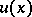to an equation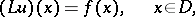(1)

which satisfies certain boundary conditions on the boundaryof(or on a part of it):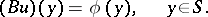(2)

As a rule, the boundary conditions relate the boundary values of the solution to its derivatives up to a certain order, i.e.is a differential operator. However, boundary conditions of other types also occur.

Given a differential equation, the question whether a specific boundary value problem should be studied is frequently settled by the concept of what is known as well-posedness. Namely, a boundary value problem is well-posed if it is solvable, and if its solution is unique and depends continuously on the data of the problem. Different types of differential equations require different well-posed boundary value problems; and conversely, well-posed boundary value problems may sometimes serve as a basis for the classification of types of differential equations.

A boundary value problem is said to be linear if the operators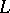andare linear, and homogeneous ifandin (1), (2) vanish. A linear boundary value problem is said to be Noetherian if: a) the homogeneous problem has a finite numberof linearly independent solutions; b) the inhomogeneous problem is solvable if and only ifandsatisfylinearly independent orthogonality conditions; and c) on the assumption that the problem is uniquely solvable, the solution depends continuously onand.

If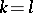, the problem is called a Fredholm problem. The differencedefines the index of the problem.

A broad range of boundary value problems for linear second-order differential equations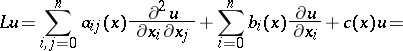(3)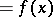falls into the category of the Poincaré problem. In this type of problem the boundary conditions are prescribed on the entire boundary, which is assumed to be an-dimensional manifold, and the boundary operatorin (2) has the form(4)

The Poincaré problem for bounded regionswith sufficiently-smooth boundaries have been thoroughly investigated in the case of uniformly-elliptic operators.

On the assumption that the coefficients of the operatorsandin (3), (4) are sufficiently smooth, and that the boundary ofis sufficiently smooth, the Poincaré problem is Noetherian if,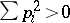, and is a Fredholm problem if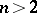,and the vector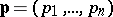is not tangent to. Investigations of the Poincaré problem in the two-dimensional case make extensive use of the theory of functions of a complex variable (see Boundary value problem, complex-variable methods).

Boundary conditions for the general elliptic equation of order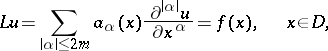(5)

may be prescribed in terms of linear differential operators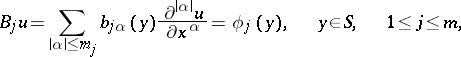(6)

of order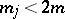, with coefficients defined on the boundaryof. Here,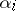is a non-negative integer,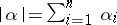andIf the operatorsand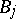satisfy the so-called complementarity conditions, then bounds for the derivatives of order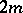of the function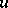satisfying the boundary conditions can be formulated (relative to an appropriate norm) in terms of the norm ofin (5) and suitable norms of the boundary functions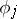in (6). Boundary value problems of this type are said to be coercive.

Another type of boundary value problems are known as mixed problems (cf. Mixed problem), in which different boundary conditions are prescribed on adjacent sections of the boundary.

Characteristic for boundary value problems of differential equations that are uniformly elliptic in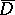is that the boundary conditions are prescribed on the entire boundary. If the operatorin (3) is elliptic in the interior of the region and parabolically degenerates on a section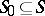, then, depending on the type of degeneracy,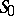can be eliminated from the specification of the boundary conditions. See also Boundary value problem, elliptic equations.

If equation (3) is not elliptic, part of the boundary may usually be eliminated from the specification of the boundary conditions. For example, given the simplest equation of parabolic type, the heat equation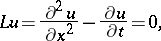the Dirichlet problem in the domainbounded by the straight lines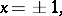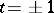amounts to the specification of boundary valueson the intervals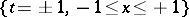and.

Boundary value problems for equations of mixed elliptic-hyperbolic type are formulated in a special manner.

Boundary value problems occupy an important position in the theory of analytic functions. Letbe a piecewise-smooth curve in the plane, i.e. a union of a finite number of simple oriented arcs. The linear conjugation problem consists in determining a function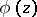, analytic outside, having limit values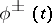,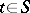, from both sides ofand satisfying the boundary condition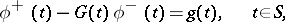where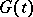andare given functions. The solution to this problem can be specified in explicit form with the help of representations of analytic functions by Cauchy-type integrals, subject to certain additional assumptions concerning the functions,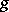and the curve. See Boundary value problems of analytic function theory.

Various methods are available for the investigation of boundary value problems. The Schwarz alternating method, Poincaré's related balayage method and the Perron method are based on an application of the maximum principle. A solution using integral equations is based on various integral representations of the solutions. Among functional methods is the investigation of boundary value problems using a priori estimates. The theory of distributions is widely applied. In practical applications, various finite-difference methods are quite frequently used.